Examples

Chapter 12 Class 12 Linear Programming (Term 1)
Serial order wise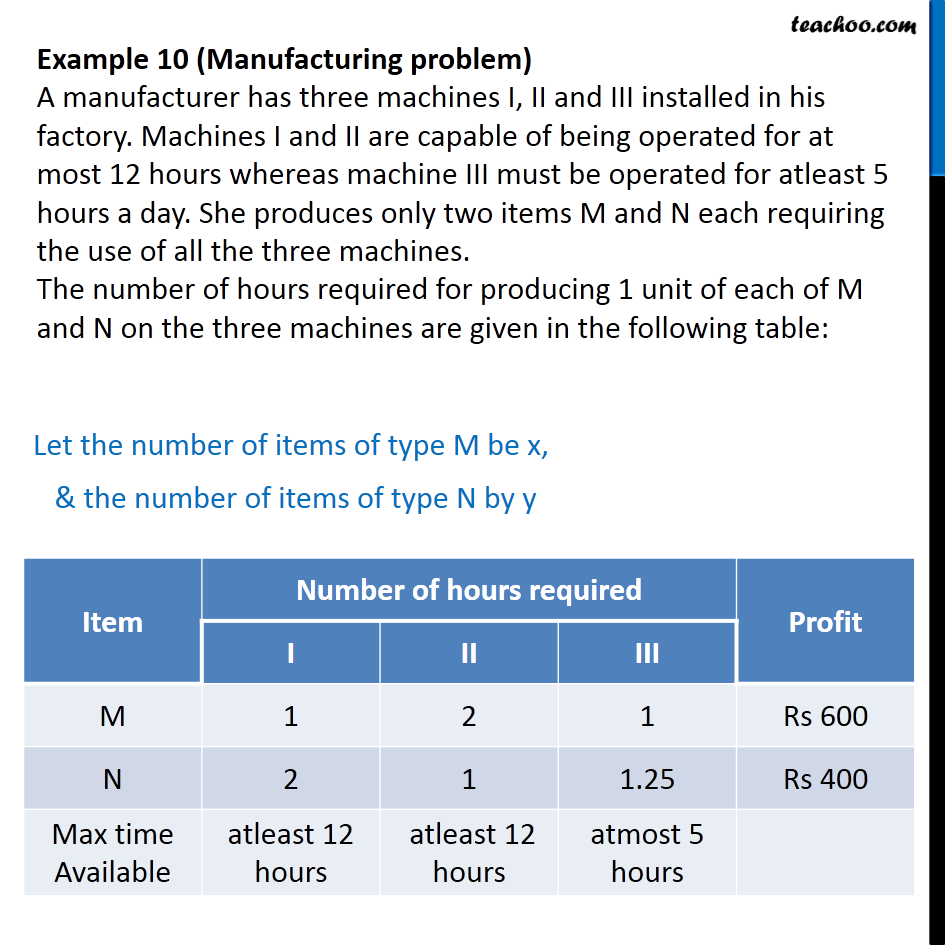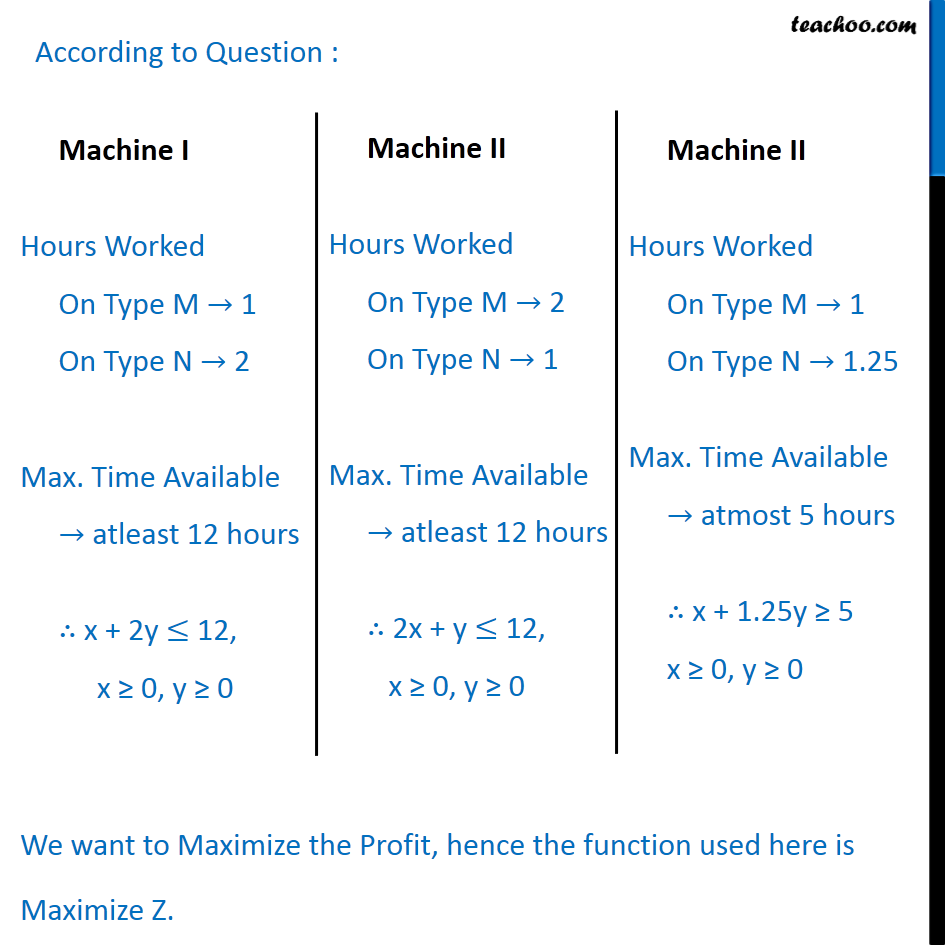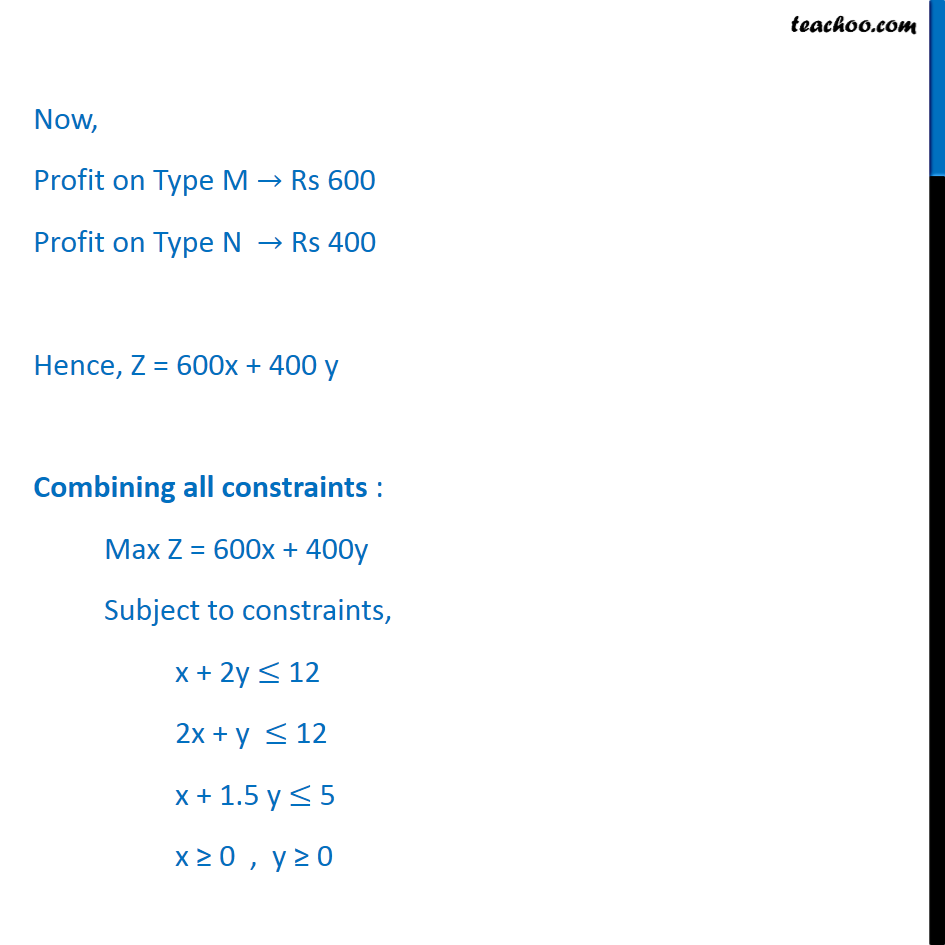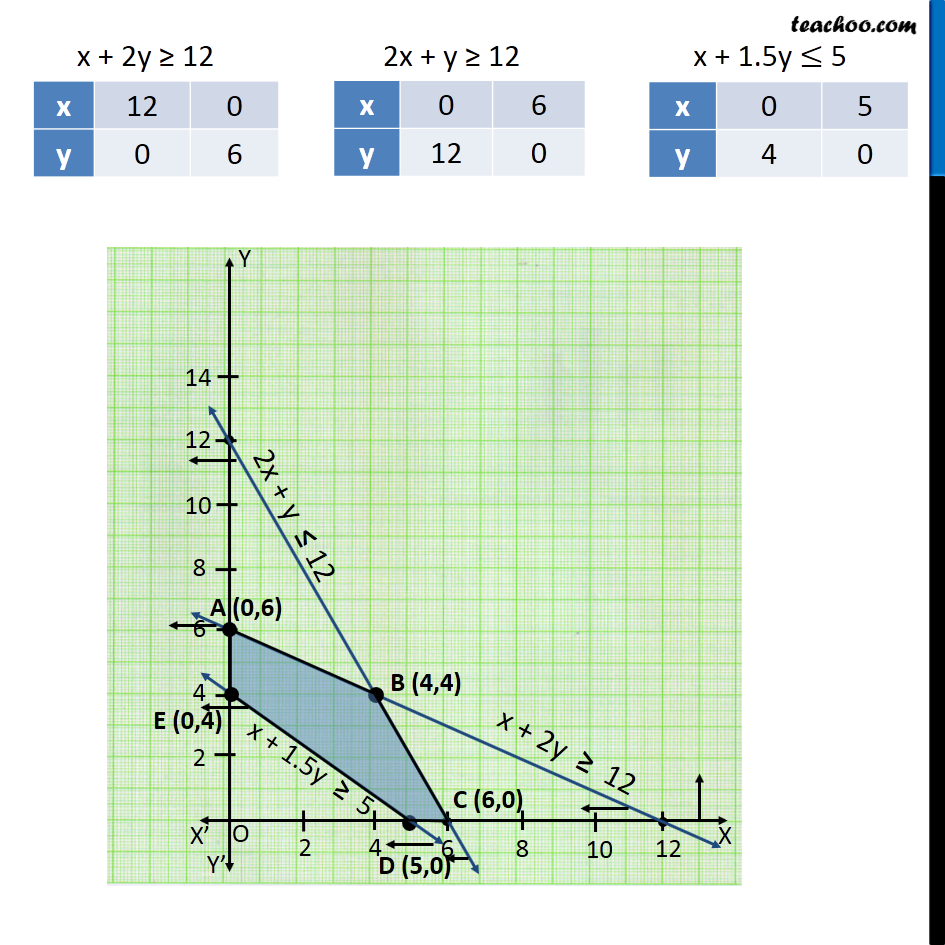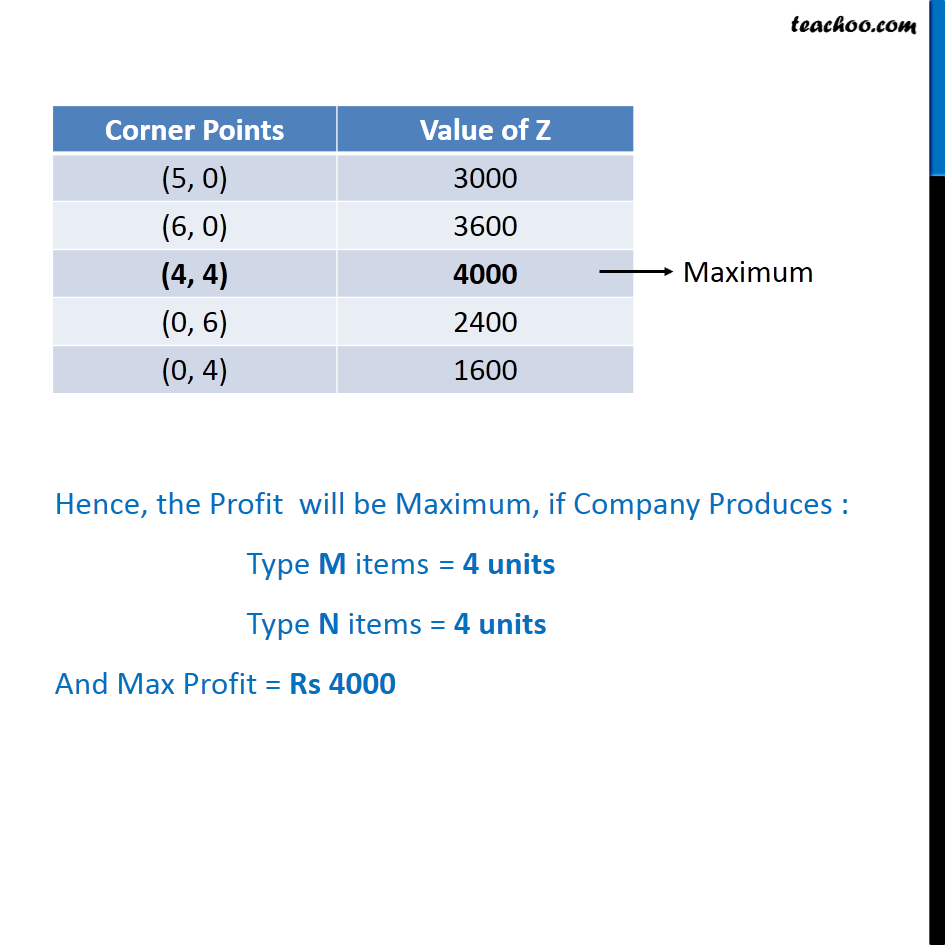### Transcript

Example 10 (Manufacturing problem) A manufacturer has three machines I, II and III installed in his factory. Machines I and II are capable of being operated for at most 12 hours whereas machine III must be operated for atleast 5 hours a day. She produces only two items M and N each requiring the use of all the three machines. The number of hours required for producing 1 unit of each of M and N on the three machines are given in the following table: Let the number of items of type M be x, & the number of items of type N by y According to Question : Now, Profit on Type M → Rs 600 Profit on Type N → Rs 400 Hence, Z = 600x + 400 y Combining all constraints : Max Z = 600x + 400y Subject to constraints, x + 2y ≤ 12 2x + y ≤ 12 x + 1.5 y ≤ 5 x ≥ 0 , y ≥ 0